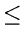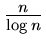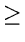Next:9.7.2 Algorithm 2Up:9.7 Order StatisticsPrevious:9.7 Order Statistics

## 9.7.1 Algorithm 1

One can arrange the n keys into a heap and pick the kth largest in k steps, where each step takes logarithmic time in the number of elements of the heap.

Since the construction of initial heap can be accomplished in worst case O(n)time, the worst case complexity of this algorithm is:

O(n + klog n)

Therefore if kor k, the above algorithm will have O(n) worst case complexity.

eEL,CSA_Dept,IISc,Bangalore